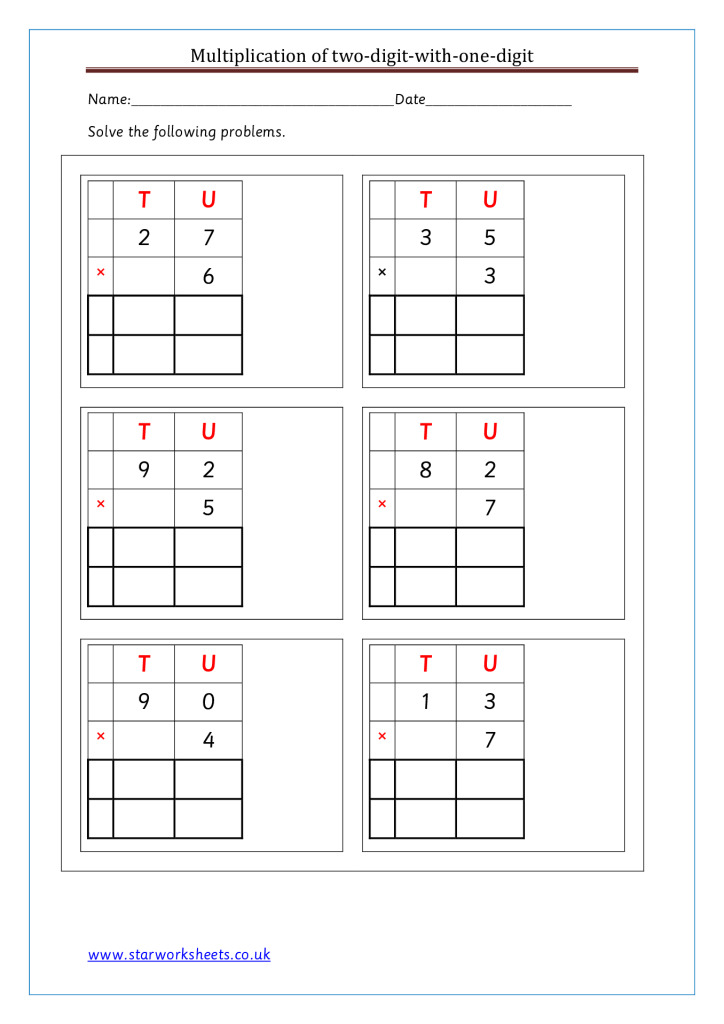# Year 4## Year 4-Multiplication of 3-digit-with 1-digit number worksheet.

Year 4-Multiplication of 3-digit-with 1-digit number worksheet. Year 4-Multiplication of 3-digit-with 1-digit number worksheet. Pupils should multiply two-digit and three-digit numbers by a one-digit number… Read More »Year 4-Multiplication of 3-digit-with 1-digit number worksheet.## Year 4 Multiplication of 2-digit number with 1-digit number worksheet.

Year 4 Multiplication of 2-digit number with 1-digit number worksheet. Year 4 Multiplication of 2-digit number with 1-digit number worksheet. Pupils should multiply two-digit and… Read More »Year 4 Multiplication of 2-digit number with 1-digit number worksheet.